# Problems on Ordinary Differential Equations using Euler, Runge-Kutta – C PROGRAMMING

I have already showed you guys how to solve an ODE using Euler Method and Runge-Kutta method.

In this post I am posting some problems on ODE with their solutions. These will help in building a better understanding of the concept and show some real-time applications in Physics.

Reference: The problems are from the Computer Programming and Numerical Analysis Manual by Dr. Shobhit Mahajan(University of Delhi).

### Prob.1.

For the differential equation$\frac{dy}{dx}=x+y \hspace{2cm}; y(0)=1$
tabulate$y(x)$ for$1\leq x\leq 5$ at intervals of 0.1 for different choices of the step size h (h =0.01; 0.005; 0.002; 0.0001), along with the analytic solution. Use all the three methods for their
comparative study. Note that though the tabulation is required between x = 1 and x = 5 only, the process of solving the equation has to begin from x = 0, since the initial condition is prescribed at that point. Also notice that tabulation has to be done at intervals of 0.1 only though the step size, h, is much smaller than that.

### Sol.

#### Code:

/************************************
************ODE PROBLEM 1************
************************************/
#include<stdio.h>
#include<math.h>
double f(double x, double y){
return x+y;
}
double euler(double f(double x, double y), double x0, double y0, double x, double h){
double y;
while(fabs(x-x0)>0.0000000001){
y=y0+h*f(x0,y0);
y0=y;
x0=x0+h;
}
return y;
}
double RK1(double f(double x, double y), double x0, double y0, double x, double h){
double y,k1,k2;
while(fabs(x-x0)>0.0000000001){
k1=h*f(x0,y0);
k2=h*f(x0+h/2.0,y0+k1/2.0);
y=y0+k2;
y0=y;
x0=x0+h;
}
return y;
}

double RK2(double f(double x, double y), double x0, double y0, double x, double h){
double y,k1,k2,k3,k4;
while(fabs(x-x0)>0.0000000001){
k1=h*f(x0,y0);
k2=h*f(x0+h/2.0,y0+k1/2.0);
k3=h*f(x0+h/2.0,y0+k2/2.0);
k4=h*f(x0+h,y0+k3);
y=y0+1/6.0*(k1+2*k2+2*k3+k4);
y0=y;
x0=x0+h;
}
return y;
}
main(){
double x0,y0,x,y,h;
printf("Enter the initial values of x and y:\nx0 = ");
scanf("%lf",&x0);
printf("y0 = ");
scanf("%lf",&y0);
printf("Enter the step-width:\nh = ");
scanf("%lf",&h);
printf("x\t\tEuler(y)\tRK1(y)\t\tRK2(y)\n");
printf("______________________________________________________\n");
for(x=1;x<=5;x=x+0.1){
printf("%lf\t",x);
y=euler(f,x0,y0,x,h);
printf("%lf\t",y);
//printf("%lf\t%lf\t%lf\t%lf\n",x0,y0,x,h);
y=RK1(f,x0,y0,x,h);
printf("%lf\t",y);
y=RK2(f,x0,y0,x,h);
printf("%lf\n",y);
}

}


### Output: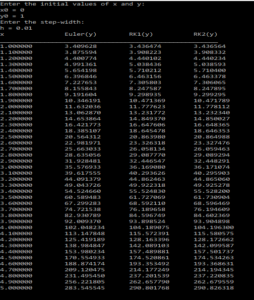### Prob.2.

The ODE describing the motion of a pendulum is$\theta''=-\sin{\theta}$
The pendulum is released from rest at an angular displacement$\alpha$ i.e.$\theta (0)=\alpha$; $\theta'(0) = 0$. Use the RK4 method to solve the equation for$\alpha= 0.1; 0.5 and 1.0$ and plot  as a function of time in the range$0\leq t \leq 8\pi$. Also plot the analytic solution valid in the small$\theta$approximation ($\sin{\theta}=\theta$).

### Sol.

#### Code:

#include<stdio.h>
#include<math.h>

double dth(double t, double theta, double z){
return z;
}

double dz(double t, double theta, double z){
return -sin(theta);
}

main(){
FILE *fp=NULL;
fp=fopen("ode_2.txt","w");
double alpha=1;
double t0=0,theta0=alpha,z0=0,t,theta,z,tf=8*M_PI;
double k1,k2,k3,k4,m1,m2,m3,m4,h=0.01;
while(t<=tf){
fprintf(fp,"%lf\t%lf\t%lf\n",t0,theta0,z0);
k1=h*dth(t0,theta0,z0);
m1=h*dz(t0,theta0,z0);
k2=h*dth(t0+h/2.0,theta0+k1/2.0,z0+m1/2.0);
m2=h*dz(t0+h/2.0,theta0+k1/2.0,z0+m1/2.0);
k3=h*dth(t0+h/2.0,theta0+k2/2.0,z0+m2/2.0);
m3=h*dz(t0+h/2.0,theta0+k2/2.0,z0+m2/2.0);
k4=h*dth(t0+h,theta0+k3,z0+m3);
m4=h*dz(t0+h,theta0+k3,z0+m3);
t=t0+h;
theta=theta0+(k1+2*(k2+k3)+k4)/6.0;
z=z0+(m1+2*(m2+m3)+m4)/6.0;
t0=t;
theta0=theta;
z0=z;

}
}


### Output: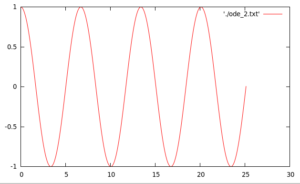### Prob.3.

A simple “prey-predator” system is modeled by the set of equations$\dot{x}=\gamma_1 x-\gamma_2 x y, \hspace{2cm}\dot{y}=\gamma_3y+\gamma_4 xy$
where$x(t)$ and$y(t)$ represent respectively the prey and predator populations as functions of time.
The term$\gamma_1 x$ tells us that the prey population grows in proportion to its own population while$-\gamma_2 xy$ says that it decreases as a result of encounters with the predators. The second equation says that the predator population decreases in proportion to its own population (to model competition for food between its members) and increases as a result of encounters with the prey (by providing food for the predators). Solve these equations for$\gamma_1 = 0.25$;$\gamma_2 = \gamma_4 = 0.01$and$\gamma_3 = 1$with the
initial values$x(0) = 100$ and successively$y(0) = 5; 10; 15; 20, and 25$. Plot$x(t)$ versus$y(t)$ for$0 \leq t \leq 20$

### Sol.

#### Code:

#include<stdio.h>
#include<math.h>
double dx(double t, double x, double y, double gamma1, double gamma2){
return gamma1*x-gamma2*x*y;
}
double dy(double t, double x, double y, double gamma3, double gamma4){
return -gamma3*y+gamma4*x*y;
}
main(){
FILE *fp=NULL;
fp=fopen("ode_3.txt","w");
double gamma1=0.25, gamma2=0.01, gamma3=1, gamma4=0.01;
double t0=0,x0=100,y0=5,x,y,t=t0,tf=20;
double h=0.01;
double k1,k2,k3,k4,m1,m2,m3,m4;
while(t<=tf){
fprintf(fp,"%lf\t%lf\t%lf\n",t0,y0,x0);
k1=h*dx(t0,x0,y0,gamma1,gamma2);
m1=h*dy(t0,x0,y0,gamma3,gamma4);
k2=h*dx(t0+h/2.0,x0+k1/2.0,y0+m1/2.0,gamma1,gamma2);
m2=h*dy(t0+h/2.0,x0+k1/2.0,y0+m1/2.0,gamma3,gamma4);
k3=h*dx(t0+h/2.0,x0+k2/2.0,y0+m2/2.0,gamma1,gamma2);
m3=h*dy(t0+h/2.0,x0+k2/2.0,y0+m2/2.0,gamma3,gamma4);
k4=h*dx(t0+h,x0+k3,y0+m3,gamma1,gamma2);
m4=h*dy(t0+h,x0+k3,y0+m3,gamma3,gamma4);
t=t0+h;
x=x0+(k1+2*(k2+k3)+k4)/6.0;
y=y0+(m1+2*(m2+m3)+m4)/6.0;
x0=x;
y0=y;
t0=t;
}

}



### Output:### Prob.4.

Solve the following differential equation:$f_1(x)\frac{d^2y}{dx^2}+f_2(x)\frac{dy}{dx}+f_3(x)y=f_4(x)$
with$y(0)=0$ at$x=0$$\frac{dy}{dx}=1$ at$x=0$
where$f_1(x)=f_2(x)=1, \hspace{1cm} f_3(x)=4x$
and,$f_4(x)=\sum_{i=0}^{10}\frac{(-1)^ix^{2i}}{(2i+1)!}$

and plot the result from x = 0 to x = 1.

### Sol.

#### Code:

/********************************************
**************PROBLEM 5.5.5******************
********************************************/
#include<stdio.h>
#include<math.h>

double f4(double x){
double t0,t1,sum,R;
t0=1;
sum=t0;
int i;
for(i=1;i<=10;i++){
R=-(x*x)/((2.0*i+1.0)*2.0*i);
t1=R*t0;
sum=sum+t1;
t0=t1;
}
return sum;
}
double dy(double x, double y, double z){
return z;
}
double dz(double x, double y, double z){
return -z-4*x*y+f4(x);
}

main(){
double x0,y0,z0,x,y,z,h;
FILE *fp=NULL;
fp=fopen("ode_4.txt","w");
printf("Enter the initial values of t, x, y, and z:\nx0 = ");
scanf("%lf",&x0);
printf("y0 = ");
scanf("%lf",&y0);
printf("z0 = ");
scanf("%lf",&z0);
printf("Enter the step-width:\nh = ");
scanf("%lf",&h);

x=10;
//BEGIN RK-4 ROUTINE
double k1,k2,k3,k4,m1,m2,m3,m4;
while(fabs(x-x0)>0.0000000001){
//fprintf(fp,"%lf\t%lf\n",x0,f4(x0));
fprintf(fp,"%lf\t%lf\t%lf\n",x0,y0,z0);

k1=h*dy(x0,y0,z0);
m1=h*dz(x0,y0,z0);
k2=h*dy(x0+h/2.0,y0+k1/2.0,z0+m1/2.0);
m2=h*dz(x0+h/2.0,y0+k1/2.0,z0+m1/2.0);
k3=h*dy(x0+h/2.0,y0+k2/2.0,z0+m2/2.0);
m3=h*dz(x0+h/2.0,y0+k2/2.0,z0+m2/2.0);
k4=h*dy(x0+h,y0+k3,z0+m3);
m4=h*dz(x0+h,y0+k3,z0+m3);

y=y0+1/6.0*(k1+2*k2+2*k3+k4);
z=z0+1/6.0*(m1+2*m2+2*m3+m4);

y0=y;
z0=z;
x0=x0+h;
}

}


### Output: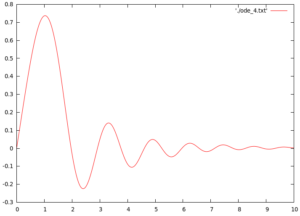### Prob.5.

Do numerical integration on the following differential equations (Lorenz equations) with integration
step size,$\Delta t=0.01$:
Plot the trajectories (after removing transients)
a) in x-y; x-z; y-z planes, and
b) in x-t; y-t; z-t planes,
for the following values of the parameter$\alpha$:
i)$\alpha$= 5.0 (fixed point solution)
ii)$\alpha$ = 50.0; 125.0; 200.0 (chaotic motion)
iii)$\alpha$ = 100.0; 150.0; 250.0 (periodic motion)
Choose any reasonable initial conditions.

### Sol.

#### Code:

/********************************************
**************PROBLEM 5.5.5******************
********************************************/
#include<stdio.h>
#include<math.h>
double dx(double t, double x, double y, double z){
return -10*(x-y);
}
double dy(double t, double x, double y, double z){
return 50*x-x*z-y;
}
double dz(double t, double x, double y, double z){
return x*y-8/3.0*z;
}
main(){
double x0,y0,z0,t0,x,y,z,t,h;
//t0=0,x0=0,y0=1,z0=0;
FILE *fp=NULL;
fp=fopen("ode_prob5.txt","w");
h=0.01;
t=10;
printf("Enter the initial values of t, x, y, and z:\nt0 = ");
scanf("%lf",&t0);
printf("x0 = ");
scanf("%lf",&x0);
printf("y0 = ");
scanf("%lf",&y0);
printf("z0 = ");
scanf("%lf",&z0);
printf("Enter the step-width:\nh = ");
scanf("%lf",&h);
double k1,k2,k3,k4,m1,m2,m3,m4,n1,n2,n3,n4;
//RK-4
while(t0<=t){
if(t0>1){
fprintf(fp,"%lf\t%lf\t%lf\t%lf\n",t0,x0,y0,z0);
}
k1=h*dx(t0,x0,y0,z0);
m1=h*dy(t0,x0,y0,z0);
n1=h*dz(t0,x0,y0,z0);
k2=h*dx(t0+h/2.0,x0+k1/2.0,y0+m1/2.0,z0+n1/2.0);
m2=h*dy(t0+h/2.0,x0+k1/2.0,y0+m1/2.0,z0+n1/2.0);
n2=h*dz(t0+h/2.0,x0+k1/2.0,y0+m1/2.0,z0+n1/2.0);
k3=h*dx(t0+h/2.0,x0+k2/2.0,y0+m2/2.0,z0+n2/2.0);
m3=h*dy(t0+h/2.0,x0+k2/2.0,y0+m2/2.0,z0+n2/2.0);
n3=h*dz(t0+h/2.0,x0+k2/2.0,y0+m2/2.0,z0+n2/2.0);
k4=h*dx(t0+x0,x0+k3,y0+m3,z0+n3);
m4=h*dy(t0+x0,x0+k3,y0+m3,z0+n3);
n4=h*dz(t0+x0,x0+k3,y0+m3,z0+n3);

x=x0+1/6.0*(k1+2*k2+2*k3+k4);
y=y0+1/6.0*(m1+2*m2+2*m3+m4);
z=z0+1/6.0*(n1+2*n2+2*n3+n4);

x0=x;
y0=y;
z0=z;
t0=t0+h;
}
}


### Output: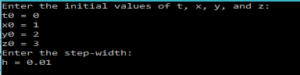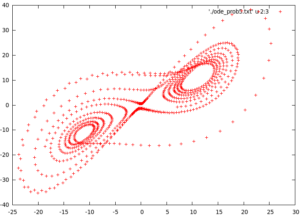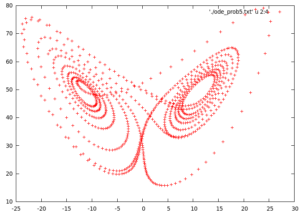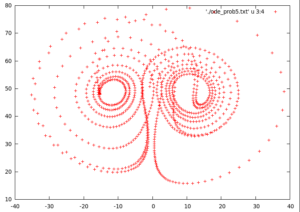### Prob.6.

To plot the bifurcation diagram for the logistic map:
A difference equation is a particular form of recurrence relation which is derived from a differential
equation. Consider a difference equation$x_{n+1}=\alpha x_n (1-x_n), \hspace{1cm} x_0\in [0,1]$
Here$\alpha$ is a parameter$\alpha \in [0,4]$. Choose a single initial value$x0$ of x in the range given for x. This can be any value EXCLUDING 0; 1 and 0.5. For this value of x0, solve the difference equation for various values of in the range given, taking$\alpha=0.05$. Thus you will have 100 values of. For the solution to the equation for each , remove the first$10^4$ iterations since these are transients.
Keep the next 100 iterations for each and plot a graph between x and .

### Sol.

#### Code:

#include<stdio.h>
#include<math.h>
double xn1(double alpha, double xn){
return alpha*xn*(1-xn);
}
main(){
FILE *fp=NULL;
fp=fopen("ode_6.txt","w");
double alpha,x=0.1,x1;
//for(x=0.1;x<=1;x=x+0.1){
if(x!=0&&x!=0.5&&x!=1){
for(alpha=0;alpha<=4;alpha=alpha+0.05){
int i=1;
x1=xn1(alpha,x);
do{
if(i>=10000){
fprintf(fp,"%lf\t%lf\n",xn1(alpha,x1),alpha);
}
x1=xn1(alpha,x1);
i++;
}while(i<=10100);
}
}
//}
}


### Output: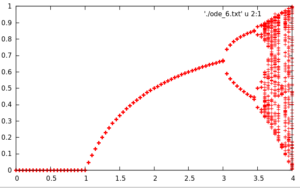Reference: The problems are from the Computer Programming and Numerical Analysis Manual by Dr. Shobhit Mahajan(University of Delhi).[wpedon id="7041" align="center"]